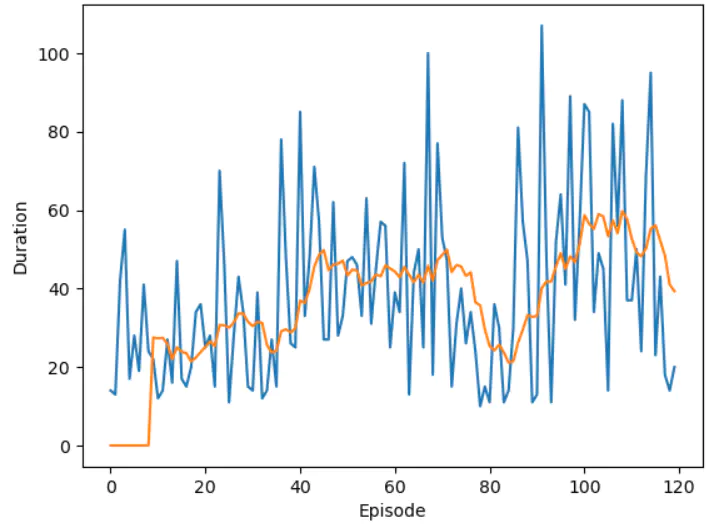# 深度强化学习DQN详解CartPole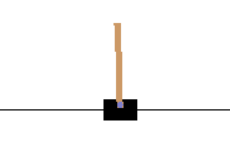``````import gym
import math
import random
import numpy as np
import matplotlib
import matplotlib.pyplot as plt
from collections import namedtuple
from itertools import count
from PIL import Image
from IPython import display

import torch
import torch.nn as nn
import torch.optim as optim
import torch.nn.functional as F
import torchvision.transforms as T
``````

``````env = gym.make('CartPole-v0')
``````

``````env
``````

<TimeLimit<CartPoleEnv<CartPole-v0>>>

``````env._max_episode_steps
``````

200

``````env.unwrapped
``````

gym.envs.classic_control.cartpole.CartPoleEnv

``````env.state
``````

array([0.00884328, 0.04488215, 0.00412898, 0.0128024 ])

``````from gym.utils import seeding
np_random, seed = seeding.np_random(None)
np_random.uniform(low=-0.05, high=0.05, size=(4,))
``````

``````env.action_space.n
``````

2

env.step(0) ：小车向左
env.step(1) ：小车向右

``````env.reset()
for t in count():
env.render()
leftOrRight = random.randrange(env.action_space.n)
_, reward, done, _ = env.step(leftOrRight)
if done:
break
``````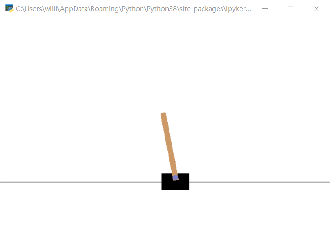``````world_width = env.x_threshold * 2
``````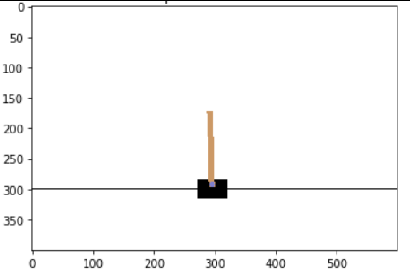400X600小车有效世界

``````scale = screen_width / world_width
``````

``````def get_cart_location(screen_width):
#世界的总长度
world_width = env.x_threshold * 2
#世界转屏幕系数 : world_unit * scale = screen_unit
scale = screen_width / world_width
#世界中点在屏幕中间，所以偏移屏幕一半
return int(env.state * scale + screen_width / 2.0)
``````

1. env = gym.make() 每个env有自己的绘制窗口
2. 环境需要初始化env.reset()
3. env.render()会打开一个绘制窗口，绘制当前状态
4. 每次env.step()会更新状态
5. 用完以后需要调用env.close()关闭绘制窗口

render有一个参数，如果指定为 mode='rgb_array'时，不但弹窗渲染，还会返回当前窗口的像素值。整个开发过程，env自己的窗口都会一只存在，不用管它，每次render()它就会刷新，刷新完又“死”了。如果想随时关掉，可以用close()，下次render()会自动打开。

``````env.reset()
screen = env.render(mode='rgb_array')
screen.shape
``````

(400, 600, 3)

``````plt.title('init state')
plt.imshow(screen)
``````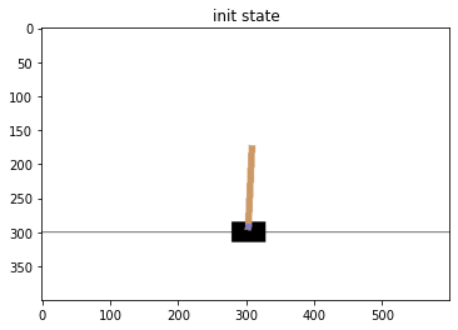``````def CutScreen(screen):
Scr2 = screen.transpose((2, 0, 1))
``````

``````    ScrCut = Scr2[:, int(screen_height*0.4):int(screen_height * 0.8)]
``````

``````    view_width = int(screen_width * 0.6)
half_view_width = view_width // 2
``````

``````    cart_location = get_cart_location(screen_width)

if cart_location < half_view_width:
#太靠左了，左边没有30%空间，则从最左侧截取  [:half_view_width)
slice_range = slice(view_width)

elif cart_location > (screen_width - half_view_width):
#太靠右了，同理 [-half_view_width:)
slice_range = slice(-view_width, None)

else:
#左右两侧都有空间，则截小车在中间 [-half_view_width: +half_view_width)
slice_range = slice(cart_location - half_view_width, cart_location + half_view_width)

#最后将图像X轴截了
ScrCut = ScrCut[:, :, slice_range]
return ScrCut
``````

C0Y1X2
Y1X2C0
``````CS = CutScreen(screen)
CS = CS.transpose((1, 2, 0))
plt.imshow(CS)
``````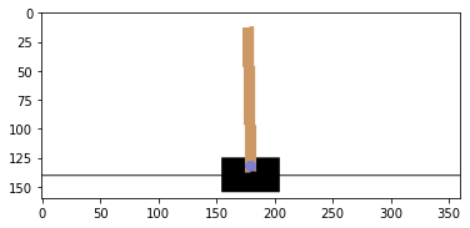``````resize = T.Compose([T.ToPILImage(),
T.Resize(40, interpolation=Image.CUBIC),
T.ToTensor()])
``````

``````device = torch.device("cuda" if torch.cuda.is_available() else "cpu")
device
``````

device(type='cuda')

``````def get_screen():
screen = env.render(mode='rgb_array')
screen = CutScreen(screen)
``````

``````    screen = torch.from_numpy(np.float32(screen)/255)
``````

``````y = x.view('float32')
``````

ValueError: To change to a dtype of a different size, the array must be C-contiguous

``````screen.flags
``````

C_CONTIGUOUS : False
F_CONTIGUOUS : False
……

``````    screen = np.ascontiguousarray(screen, dtype=np.float32) / 255
screen = torch.from_numpy(screen)
``````

N表示batch数
C表示channel数
Y，X表示图片的高和宽。

``````    return resize(screen).unsqueeze(0).to(device)
``````

unsqueeze()的作用是在n维之前增加一个维度，这里是在0维之前增加一个维度，增加前 screen尺寸是

torch.Size([3, 40, 90])

torch.Size([1, 3, 40, 90])

``````scr = get_screen().cpu().squeeze(0).permute(1, 2, 0).numpy()

plt.figure()
plt.imshow(scr)
plt.title('Example extracted screen')
plt.show()
``````

40 X 90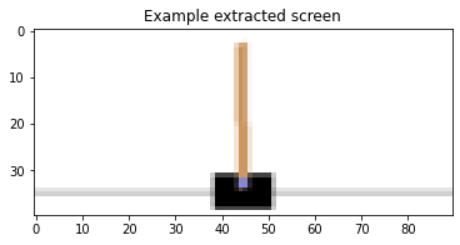OK。图像处理完了，接下来要定义网络，训练网络了。

# 二、 卷积网络和训练

python几处值得关注的用法（连接）

``````def conv2d_size_out(size, kernel_size = 5, stride = 2):
return (size - (kernel_size - 1) - 1) // stride  + 1

class DQN(nn.Module):
def __init__(self, h, w, outputs):
super(DQN, self).__init__()
self.conv1 = nn.Conv2d(3, 16, kernel_size=5, stride=2)
self.bn1 = nn.BatchNorm2d(16)
self.conv2 = nn.Conv2d(16, 32, kernel_size=5, stride=2)
self.bn2 = nn.BatchNorm2d(32)
self.conv3 = nn.Conv2d(32, 32, kernel_size=5, stride=2)
self.bn3 = nn.BatchNorm2d(32)

convw = conv2d_size_out(conv2d_size_out(conv2d_size_out(w)))
convh = conv2d_size_out(conv2d_size_out(conv2d_size_out(h)))
linear_input_size = convw * convh * 32

# Called with either one element to determine next action, or a batch
# during optimization. Returns tensor([[left0exp,right0exp]...]).
def forward(self, x):
x = F.relu(self.bn1(self.conv1(x)))
x = F.relu(self.bn2(self.conv2(x)))
x = F.relu(self.bn3(self.conv3(x)))
``````

• Conv 3通道 16通道
• Conv 16通道 32通道
• Conv 32通道 32通道
• Linear 512节点 2节点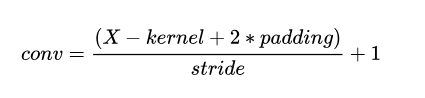conv 为某维度上卷积后的尺寸，X为卷积前的尺寸。

``````(W - kernel_size + 2 * padding ) // stride + 1
``````

``````(size - kernel_size) // stride  + 1
``````

``````def conv2d_size_out(size, kernel_size = 5, stride = 2):
return (size - (kernel_size - 1) - 1) // stride  + 1
``````

``````        convw = conv2d_size_out(conv2d_size_out(conv2d_size_out(w)))
convh = conv2d_size_out(conv2d_size_out(conv2d_size_out(h)))
linear_input_size = convw * convh * 32
``````

``````net = DQN(40, 90, 2).to(device)
scr = get_screen()
net(scr)
``````

OK，返回两个值。

``````EPS_START = 0.9 # 概率从0.9开始
EPS_END = 0.05  #     下降到 0.05
EPS_DECAY = 200 #     越小下降越快
steps_done = 0 # 执行了多少步
``````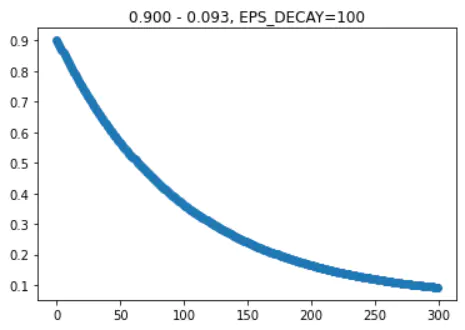100时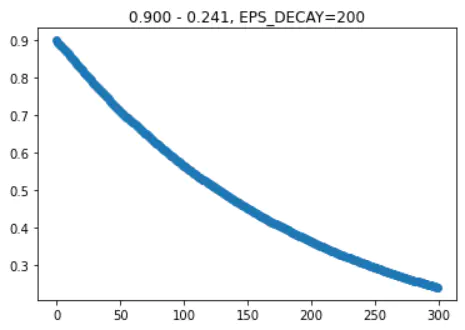200时

``````def select_action(state):
global steps_done
sample = random.random() #[0, 1)
#epsilon greedy policy。EPS_END 加上额外部分，steps_done 越小，额外部分越接近0.9
eps_threshold = EPS_END + (EPS_START - EPS_END) * math.exp(-1. * steps_done / EPS_DECAY)
steps_done += 1
if sample > eps_threshold:
#选择使用网络来做决定。max返回 0:最大值和 1:索引
return policy_net(state).max(1).view(1, 1)
else:
#选择一个随机数 0 或 1
``````

pytorch 的 tensor.max() 返回所有维度的最大值及其索引，但如果指定了维度，就会返回namedtuple，包含各维度最大值及索引 (values=..., indices=...) 。

max(1) 只取了索引值，也可以用 max(1).indicesview(1,1) 把数值做成[] 的二维数组形式。为何返回一个二维 [] ? 这是因为后面要把所有的state用torch.cat() 合成batch（cat()说明连接）

``````    return policy_net(state).max(1).view(1, 1)
# return 0 if value > value else 1
``````

``````for t in count():
# 1. 获取屏幕 1
last_screen = get_screen()
# 2. 选择行为、步进
action = select_action(state)
_, reward, done, _ = env.step(action)
# 3. 获取屏幕 2
current_screen = get_screen()
# 4. 计算差别 2-1
state = current_screen - last_screen
# 5. 优化网络
optimize_model()
``````

• 上边两个分别是step0和step1原图
• 中间灰色图是差值部分，蓝色是少去的部分，棕色是多出的部分
• 下面两图是原始图覆盖差值图，step0将完全复原为step1，step1则多出部分颜色加强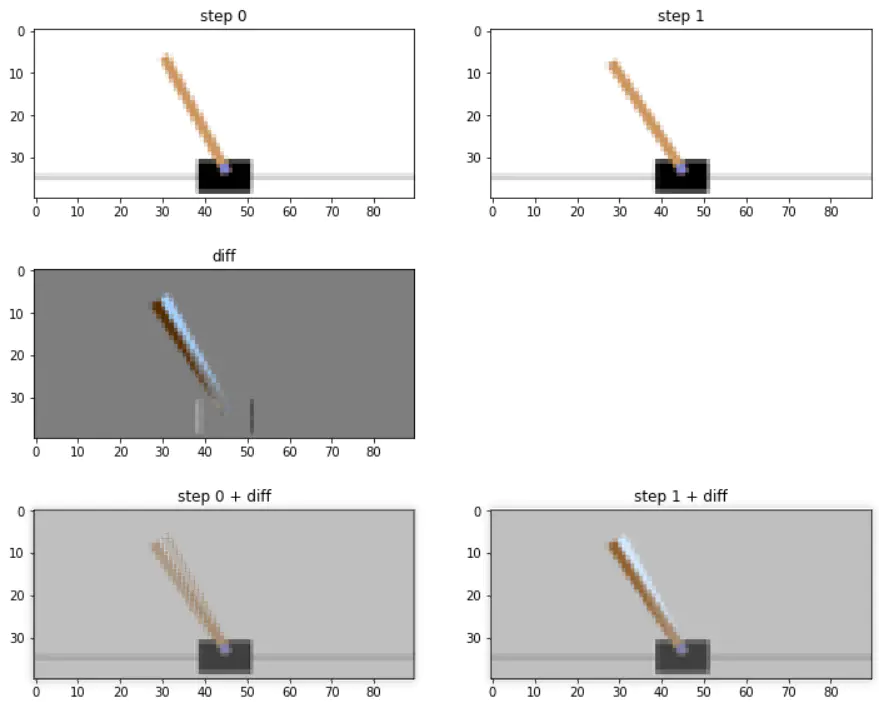``````num_episodes = 50
TARGET_UPDATE = 10

for i_episode in range(num_episodes):
env.reset()
last_screen = get_screen()
current_screen = get_screen()
state = current_screen - last_screen

# [0, 无限) 直到 done
for t in count():
action = select_action(state)
_, reward, done, _ = env.step(action.item())
reward = torch.tensor([reward], device=device)
last_screen = current_screen
current_screen = get_screen()
next_state = None if done else current_screen - last_screen
// 保存 state, action, next_state, reward 到列表 memory

state = next_state
optimize_model()

if done:
break

``````

1. 从memory列表里选取n个 （state, action, next_state, reward）
2. 用net获取state的 Y[0,1]（net输出为2个值），再用action选出结果y
3. 用net获取next_state获取Y'[0,1]，取最大值y'。如果state没有对应的next_state，则y'=0
4. 用公式算出期望y：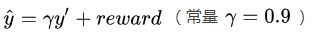5. 用smooth_l1_loss计算误差
6. 用RMSprop 反向传导优化网络